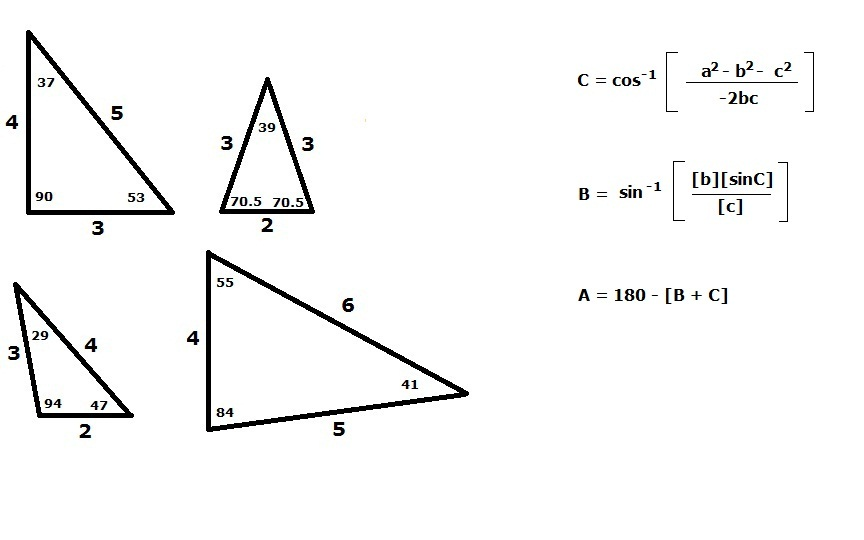Cycle I Physics
John Dewey High School
Mr. Klimetz
Geometric and Arithmetic Features of Triangles
Laboratory No. 2
Introduction. Mathematics is the principal tool applied by physicists to the solution of physics problems as well as the measurement and expression of physical phenomena. The solution to many problems in physics as well as the measurement and expression of many physical phenomena can be equally (and just as accurately) accomplished through purely graphical methods. As we will see in future exercises, vector analyses and mathematics, for example, depends greatly on our understanding of the branch of mathematics that is concerned with the features of the three-sided polygon (nee triangle), trigonometry.  The purpose of this lab is to permit you to gain a better understanding of trigonometry and its application to the graphical (as well as mathematical) solution of problems in physics by constructing a series of triangles with particular angle and side-length characteristics. This lab is also designed to sharpen and refine your skills in drafting through the application of the protractor and pencil compass.
Equipment.Metric rulerPencilEraserProtractorPencil CompassPencil SharpenerUnruled Paper

Techniques and Skills in Physical Measurement II: Trigonometry
Procedure.

I.  Using the tools provided, draft a "3:4:5" side-length ratio triangle. Construct the figure of sufficient dimensions so as to occupy as much of the paper as possible. (Miniature figures are difficult to draw and notoriously inaccurate!) You must first decide upon and draft a scale and accompanying metric scale factor before you commence drawing. A 2:3:3 triangle, for example, could be drawn as a 20 cm:30 cm:30 cm triangle with a scaling factor of one unit length equal to 10 cm. Your scaling factor must appear with each of your drafted triangles. (As a suggestion, a scaling factor of one unit length equal to 3 cm works fairly well.) Draw the longest side of your triangle first near the edge of the paper. Extend the ends of your pencil compass to the distance of one of the remaining side lengths. Place the pivot pin at the left-end of the previously-drafted line and sweep out a generous arc. Then extend the ends of your pencil compass to the distance of the remaining undrafted side length. Place the pivot pin of the compass at the right-end of the first drafted line and sweep out another arc of sufficient length so that it intersects the first arc. Then draft two lines, one extending from each opposing end of the first drafted line to the point of intersection of the arcs. You now have completed a proportional length triangle. Work carefully and slowly to ensure that your figures are accurate. (Sharp pencil points, for example, yield thin lines which increase the accuracy of your finished product.) Label each side in appropriate units. Measure and label each interior angle. Calculate the sum of the interior angles. Does the sum equal 180 degrees? Show all work next to the drafted figure.

II.  Using the tools provided, draft a "4:5:6" side-length ratio triangle. As in procedure I, construct the figure of sufficient dimensions so as to occupy as much of the paper as possible after first deciding upon and drawing a scale and accompanying metric scaling factor before drawing. Label each side in appropriate units. Measure and label each interior angle. Calculate the sum of the interior angles. Does the sum equal 180 degrees? Show all work next to the drafted figure.

III.  Using the tools provided, draft a "2:3:3" side-length ratio triangle and a "2:3:4" side-length ratio triangle following the same procedures outlined above. Don't forget to label each side in appropriate units and measure and label each interior angle. Calculate the sum of the interior angles of each triangle. Do the sums equal 180 degrees? Show all work next to each drafted figure.

IV.  Mathematical Check. Using either the Pythagorean Theorem, SOH-CAH-TOA, the Law of Sines, or the Law of Cosines, demonstrate mathematically that each figure (as constructed and measured) is indeed correct by solving for each interior angle and comparing with your measured values. Show all work on the back of or adjacent to each drafted figure.
Questions.

1.  Can we ever measure any physical feature of a natural system exactly? Why or why not? Explain how this exercise contributed to your conclusion.
2.  Can we ever draw any physical feature of a natural system exactly? Why or why not? Explain how this exercise contributed to your conclusion.
3.  Define, in your own words, the meaning and value of measurement.
4.  Explain how we could have achieved greater accuracy in our exercises.
5.  An airplane points due north, traveling through the Earth's atmosphere from the power provided by its engines. The Earth's atmosphere around the plane is moving due east, powered by the heating of the Earth by the Sun. The plane and atmosphere are moving at the same speed, but in different directions. Using this information, your knowledge of triangles, and your common sense, what will be the actual direction of flight of the airplane? Make a sketch to support your claim.
6.  Sketch a "1:2:3" triangle.Solutions.